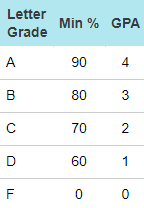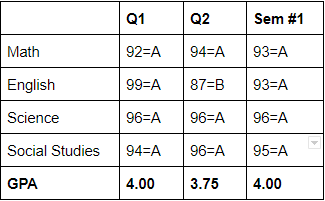# Why is summary GPA not an average of regular grading period GPAs?

Sometimes, our admins are surprised to find a student’s summary grading period GPA is not the average of the student’s regular grading period GPAs. This is a common misconception with how summary grading periods are calculated. In this article, we will discuss:

## How summary grading period GPAs are calculated

Summary grading period GPAs are calculated from summary grading period grades. As such, they have no relation to regular grading period GPAs. This is illustrated by the example below:

### Example of a Summary Grading Period GPA Calculation

At this school, students are graded on a simple A-B-C-D-F grading scale, pictured below.Elena received straight As in Q1, but in Q2, she got a B in English. Despite this setback, she still received an A for the semester (in this case, a 99 in Q1 and an 87 in Q2 average out to a 93 for the semester).In this chart, you can see that the GPA is calculated for each grading period separately. The Sem #1 GPA is calculated based solely on the Sem #1 report card grades. The B in Q2 has no effect. The Sem #1 GPA is not an average of the Q1 and Q2 GPAs.

This is a simple example, but the idea holds true in every configuration of grading periods. It can also work in reverse, with a lower Sem #1 GPA than you would expect based on the quarterly GPAs.